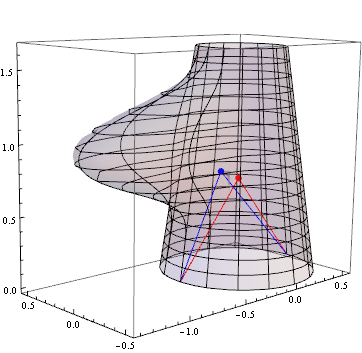# Calculating the COM of a 3D Surface of Revolution

My current research at Rutgers focuses on identifying the cognitive mechanisms that are employed when perceiving the shape and structure of 3D objects. One of the little problems that I had to overcome was finding out how to calculate the center of mass/gravity of a 3D surface of revolution analytically. Specifically, I needed to calculate the COM for conical frustums with and without an attached part (which was also analytically generated).Unfortunately, it wasn’t as easy to derive as I assumed, but the steps toward that end are pretty straightforward.  After the break you can see the general equation I ultimately derived for calculating the COM in 3D-space.

A conical frustum will be defined with attributes$R_{1}$ (the top radius),$R_{2}$ (the bottom radius), and$h$ (the height of the truncated cone).

The radius of the frustum at any given height$z$ is:$r_{fr}(z)=\frac{h-z}{h} R_{1} + \frac{z}{h} R_{2}$

The area of this slice at any given height$z$ is:$A(z) = \int_0^{2\pi} \! \int_0^{r_{fr}(z)} \! r \, dr \, d\theta = \pi r_{fr}(z)^2$

Then the volume can be calculated by integrating across all heights:$V = \int_0^{h} \! A(z) , dz$

Combine these three equations together and we have:$V = \int_0^{h} \! \pi (\frac{h-z}{h} R_{1} + \frac{z}{h} R_{2})^2 \, dz = \frac{1}{3} h \pi (R_{1}^2 + R_{1} R_{2} + R_{2}^2)$

Ok, so now we have the radius, area, and volume of the conical frustum. Using this information, we can now calculate the center of mass.$COM_{x} = \frac{1}{V} \int_0^h \! \int_0^{2\pi} \! \int_0^{r_{fr}(z)} \! r^2 \cos(\theta) A(z) \, dr \, d\theta \, dz = 0$$COM_{y} = \frac{1}{V} \int_0^h \! \int_0^{2\pi} \! \int_0^{r_{fr}(z)} \! r^2 \sin(\theta) A(z) \, dr \, d\theta \, dz = 0$$COM_{z} = \frac{1}{V} \int_0^h \! z A(z) \, dz$

Doing a little substitution with$COM_{z}$:$COM_{z} = \frac{1}{V} \int_0^{h} \! z \pi (\frac{h-z}{h} R_{1} + \frac{z}{h} R_{2})^2 \, dz$$= \frac{1}{V} \frac{h^2 \pi (R_{1}^2 + 2 R_{1} R_{2} + 3 R_{2}^2)}{12}$$= \frac{h (R_{1}^2 + 2 R_{1} R_{2} + 3 R_{2}^2)}{4(R_{1}^2 + R_{1} R_{2} + R_{2}^2)}$

Note that because the conical frustum is radially symmetric around the z-axis, the COM for both x and y will be 0. This obviously will not be the case for shapes with more complex surfaces of revolution, but is a nice little proof.

Complex, you want complex??

So, we will add a simple part onto the shape (like the one in the image above), defined using both a radial function,$f_{rad}(\theta)$, and a vertical function,$f_{vert}(z)$. The functions themselves can be any analytical functions, as long as they are continuous. And frankly, it doesn’t matter for this proof, so we’ll leave them undefined for the time being.

Remember how simple the radius at any given height was? (Just to remind you:$r_{fr}(z)=\frac{h-z}{h} R_{1} + \frac{z}{h} R_{2}$). Now we need to add our radial and vertical functions, making the function dependent upon not only height, but also angle:$r_{fr}(z,\theta)=\frac{h-z}{h} R_{1} + \frac{z}{h} R_{2} + f_{vert}(z) f_{rad}(theta)$

Given that the radius is now a bit more complex, the area of this slice at any given height$z$ is now:$A(z) = \int_0^{2\pi} \! \int_0^{r_{fr}(z,\theta)} \! r \, dr \, d\theta$

Then the volume is now a bit more complicated:$V = \int_0^{h} \! \int_0^{2\pi} \! \int_0^{r_{fr}(z,\theta)} \! r \, dr \, d\theta \, dz$

And now we can calculate the COMs:$COM_{x} = \frac{1}{V} \int_0^h \! \int_0^{2\pi} \! \int_0^{r_{fr}(z,\theta)} \! r^2 \cos(\theta) (\int_0^{2\pi} \! \int_0^{r_{fr}(z,\theta)} \! r \, dr \, d\theta) \, dr \, d\theta \, dz$$COM_{y} = \frac{1}{V} \int_0^h \! \int_0^{2\pi} \! \int_0^{r_{fr}(z,\theta)} \! r^2 \sin(\theta) (\int_0^{2\pi} \! \int_0^{r_{fr}(z,\theta)} \! r \, dr \, d\theta) \, dr \, d\theta \, dz$$COM_{z} = \frac{1}{V} \int_0^h \! z (\int_0^{2\pi} \! \int_0^{r_{fr}(z,\theta)} \! r \, dr \, d\theta) \, dz$

The good news, all you really need to define is$r_{fr}(z,theta)$ and h. The bad news, pentuple integrals. Substituting all of the necessary equations, we get these monstrosities:$COM_{x} = \frac{\int_0^h \! \int_0^{2\pi} \! \int_0^{\frac{h-z}{h} R_{1} + \frac{z}{h} R_{2} + f_{vert}(z) f_{rad}(\theta)} \! r^2 \cos(\theta) (\int_0^{2\pi} \! \int_0^{\frac{h-z}{h} R_{1} + \frac{z}{h} R_{2} + f_{vert}(z) f_{rad}(\theta)} \! r \, dr \, d\theta) \, dr \, d\theta \, dz}{\int_0^{h} \! \int_0^{2\pi} \! \int_0^{\frac{h-z}{h} R_{1} + \frac{z}{h} R_{2} + f_{vert}(z) f_{rad}(\theta)} \! r \, dr \, d\theta \, dz}$$COM_{y} = \frac{\int_0^h \! \int_0^{2\pi} \! \int_0^{\frac{h-z}{h} R_{1} + \frac{z}{h} R_{2} + f_{vert}(z) f_{rad}(\theta)} \! r^2 \sin(\theta) (\int_0^{2\pi} \! \int_0^{\frac{h-z}{h} R_{1} + \frac{z}{h} R_{2} + f_{vert}(z) f_{rad}(\theta)} \! r \, dr \, d\theta) \, dr \, d\theta \, dz}{\int_0^{h} \! \int_0^{2\pi} \! \int_0^{\frac{h-z}{h} R_{1} + \frac{z}{h} R_{2} + f_{vert}(z) f_{rad}(\theta)} \! r \, dr \, d\theta \, dz}$$COM_{z} = \frac{\int_0^h \! z (\int_0^{2\pi} \! \int_0^{\frac{h-z}{h} R_{1} + \frac{z}{h} R_{2} + f_{vert}(z) f_{rad}(\theta)} \! r \, dr \, d\theta) \, dz}{\int_0^{h} \! \int_0^{2\pi} \! \int_0^{\frac{h-z}{h} R_{1} + \frac{z}{h} R_{2} + f_{vert}(z) f_{rad}(\theta)} \! r \, dr \, d\theta \, dz}$

Not very pretty, ehh? Well, in the meantime, I’ll leave the final calculations for you to work on. Suffice to say, it is a pain in the butt.

This site uses Akismet to reduce spam. Learn how your comment data is processed.